30040050060070080088090010001050110012001350140015001550160016501750180019502000220024002600280030003200

3004005006008001000120014001800×180×25001200×120×25001400×140×25001600×160×25002000×200×25002200×220×25001000×100×20001200×120×20001500×150×20001800×180×20002000×200×2000800×80×20001000×100×25002400×230×25002600×235×25002800×255×2500600×60×2500800×80×25001350×135×20001500×20003000×275×25001000×100×40001100×110×25001350×135×25001400×160×25001500×150×25001550×160×25001650×165×20001650×165×25001800×2000400×45×2500600×60×2000700×70×2000700×70×2500900×90×25001000×3000×1251000×40001200×25001200×3000×1401350×20001350×3000×1421400×3000×1601500×3000×1601550×3000×1611600×3000×1601650×3000×1651800×3000×1802000×20002000×3000×2002200×3000×2202400×3000×2402600×3000×2402800×2500×2553000×2500×275600×60×4000800×3000×80800×80×40001000×100×30001000×120×25001000×3000×1001200×120×30001200×120×40001200×135×25001350×135×30001500×150×30001500×160×25001650×165×30001800×180×30003000×200×3000300×35×2500300×35×4000400×4000400×45×4000500×4000500×55×2500500×55×4000600×4000800×120×2500800×4000800×80×30001000×20001000×25001200×20001200×3000×1201500×25001650×1650×20001650×20001800×25002000×200×30002000×25002200×20002800×25003000×2500300×2500×40400×3000×60500×3000×60600×3000×60600×60×3000700×3000×70700×70×3000800×2000900×3000×901000×125×25001000×125×30001000×130×30001000×2000×1001000×2500×1251050×105×25001050×117×25001050×25001050×3000×1171200×130×25001200×140×25001200×2000×1001350×1350×20001350×142×25001350×25001400×25001500×2000×1501550×155×25001550×25001600×165×25001600×25001650×25001650×2500×1651750×175×25001750×25001750×3000×1751800×2500×1801950×25001950×3000×1902000×2500×2002200×25002200×2500×2202400×240×25002400×25002400×2500×2402600×25002600×2500×2352600×2500×2602600×260×2500300×2000300×30×2000300×30×3000300×65×10003200×2500400×2000400×2000×40400×40×2000400×70×1000500×50×2000500×50×2500500×86×1000600×2500600×80×2500600×87×1000800×2500880×100×3000880×2500880×88×25001000×2000×751200×2000×1201200×2500×1201200×40001350×1350×30001350×2000×1451400×20001400×2000×1201500×2000×1151500×2000×1601600×20001600×2500×1601650×1650×30002800×280×2500300×1000300×30×4000300×40×2000400×1000400×4000×40400×40×1000400×40×3000400×40×4000500×1000500×2000500×2000×45500×2000×50500×50×1000500×50×3000500×50×4000600×1000600×2000600×2000×50600×2000×60600×4000×50600×50×4000800×100×2500800×100×3000800×2000×65800×2000×80

2020201920182017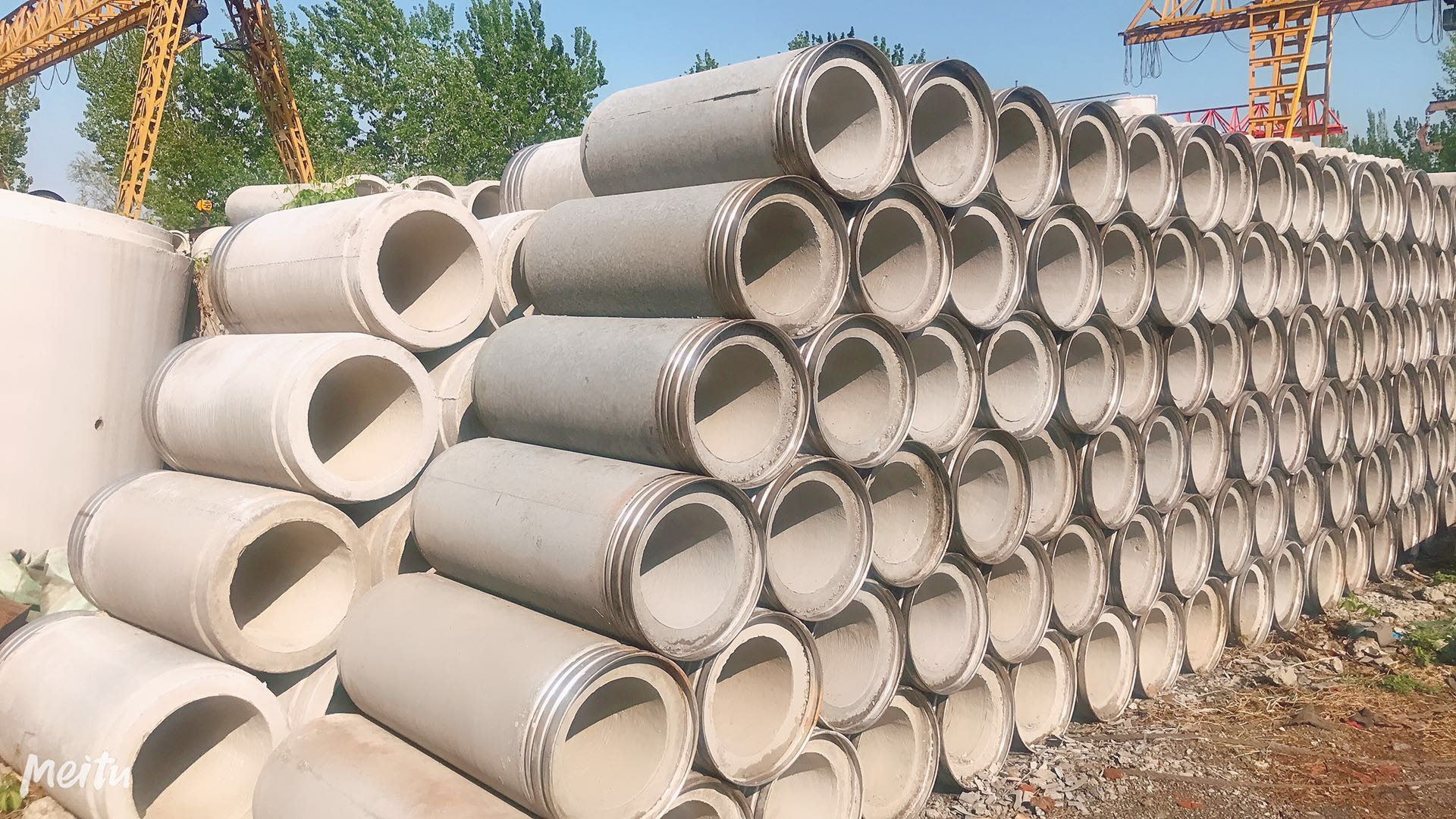m

13%
2020/10/28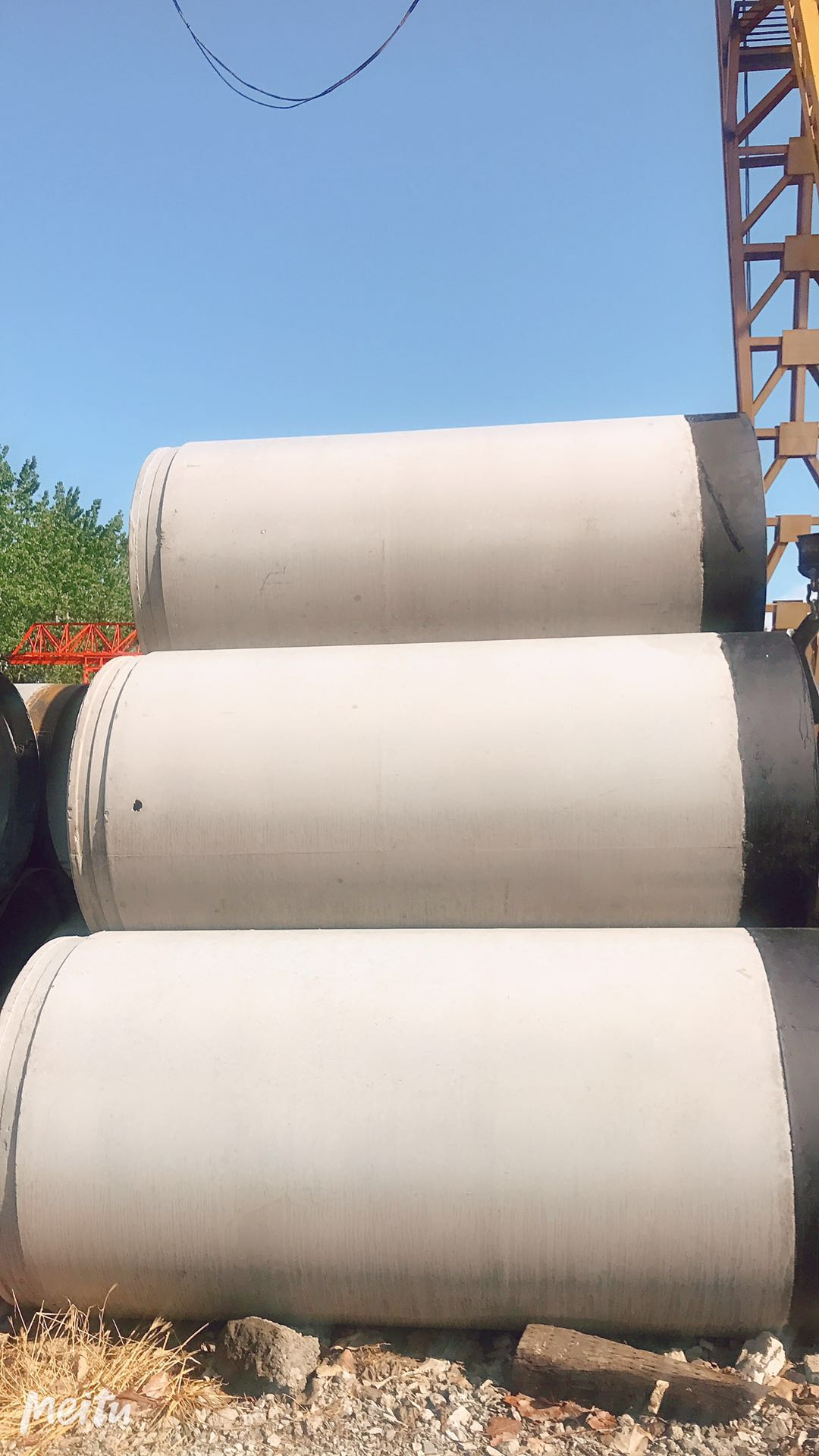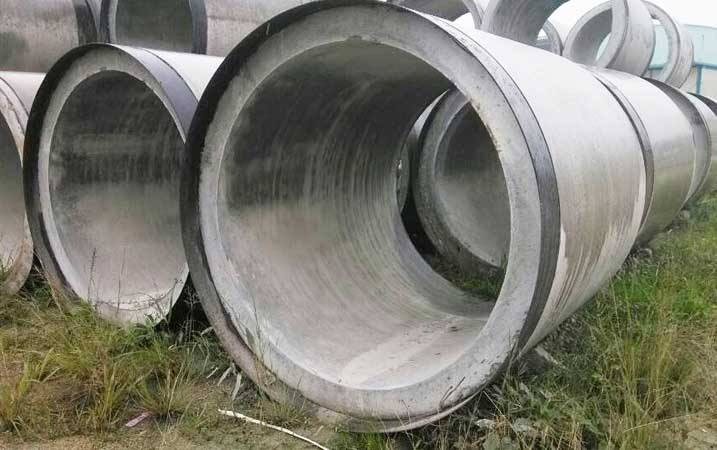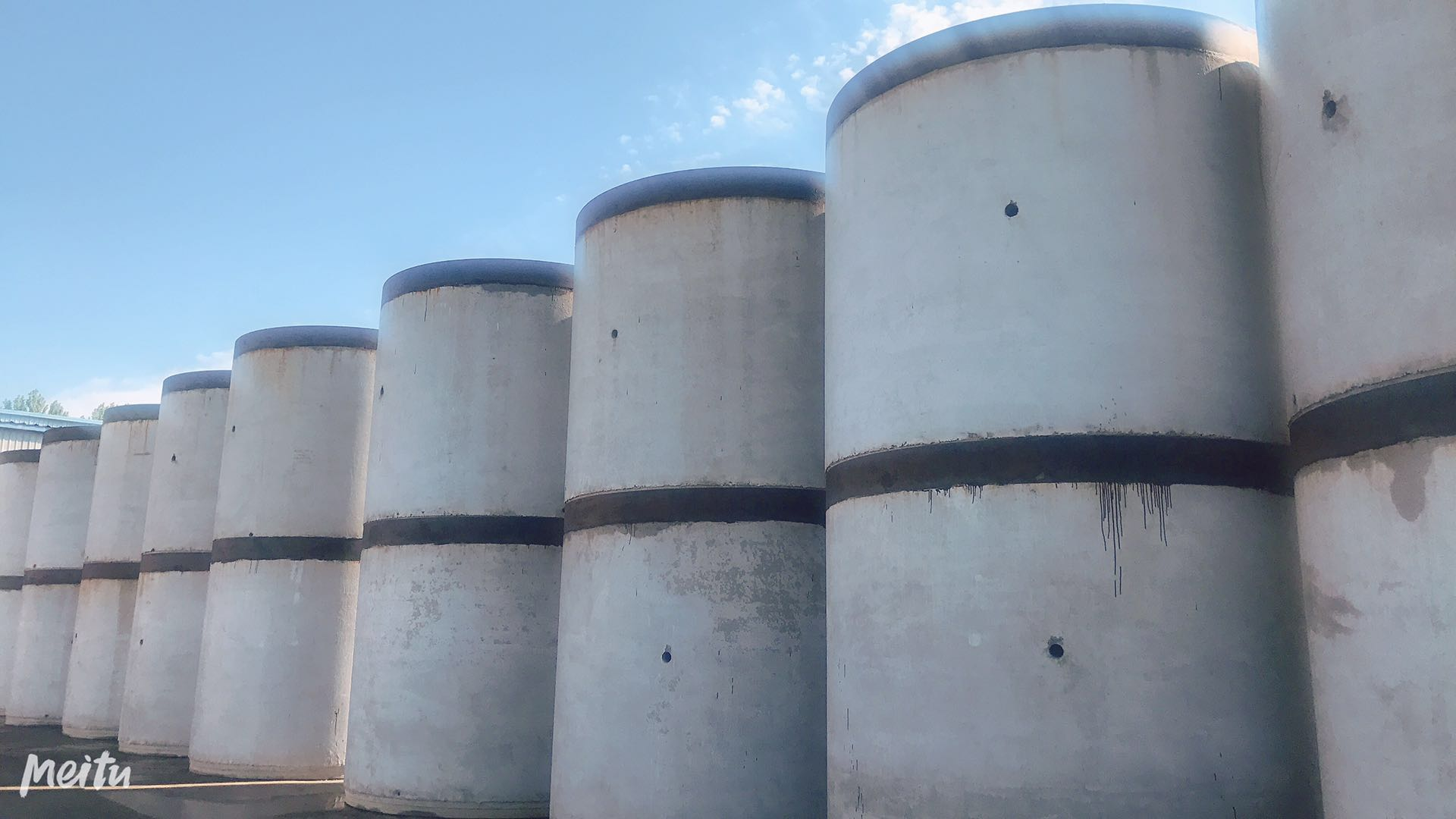m

13%
2020/10/28m

13%
2020/10/28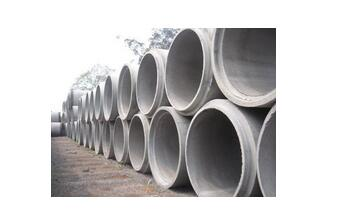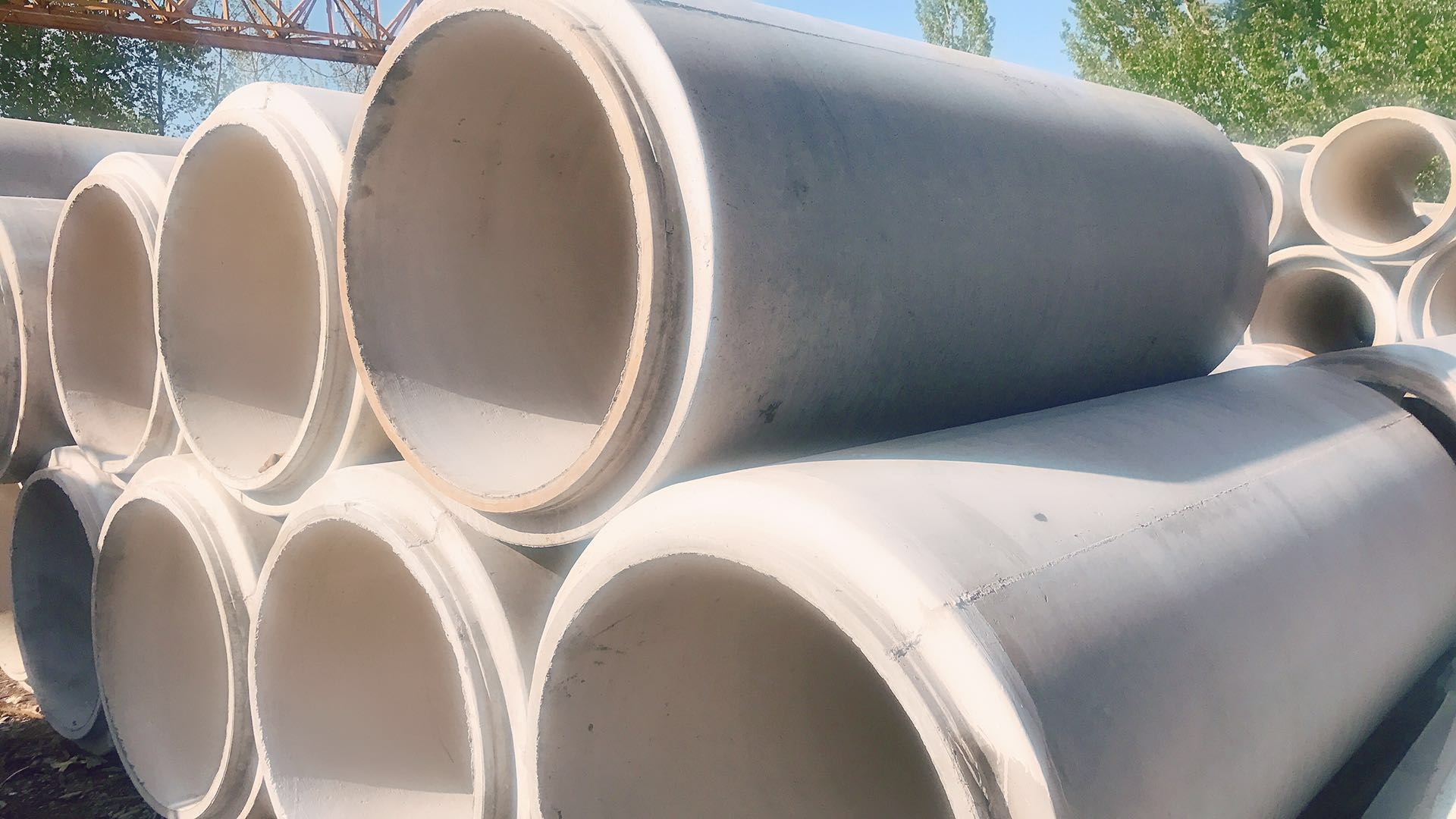m

13%
2020/10/28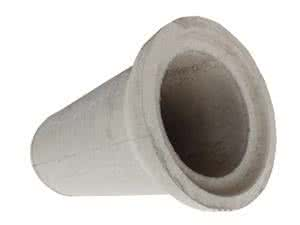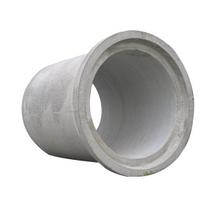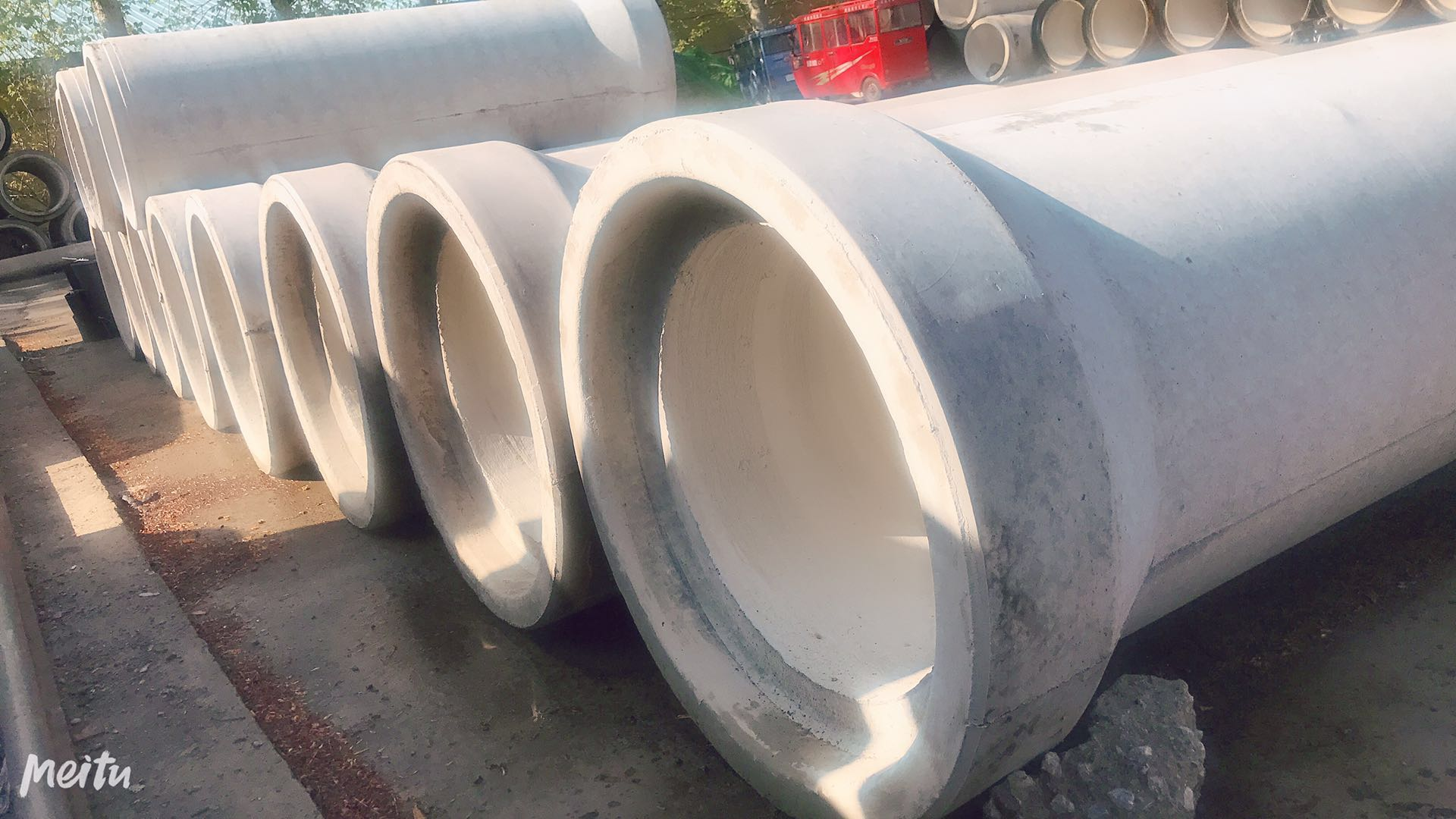m

13%
2020/10/28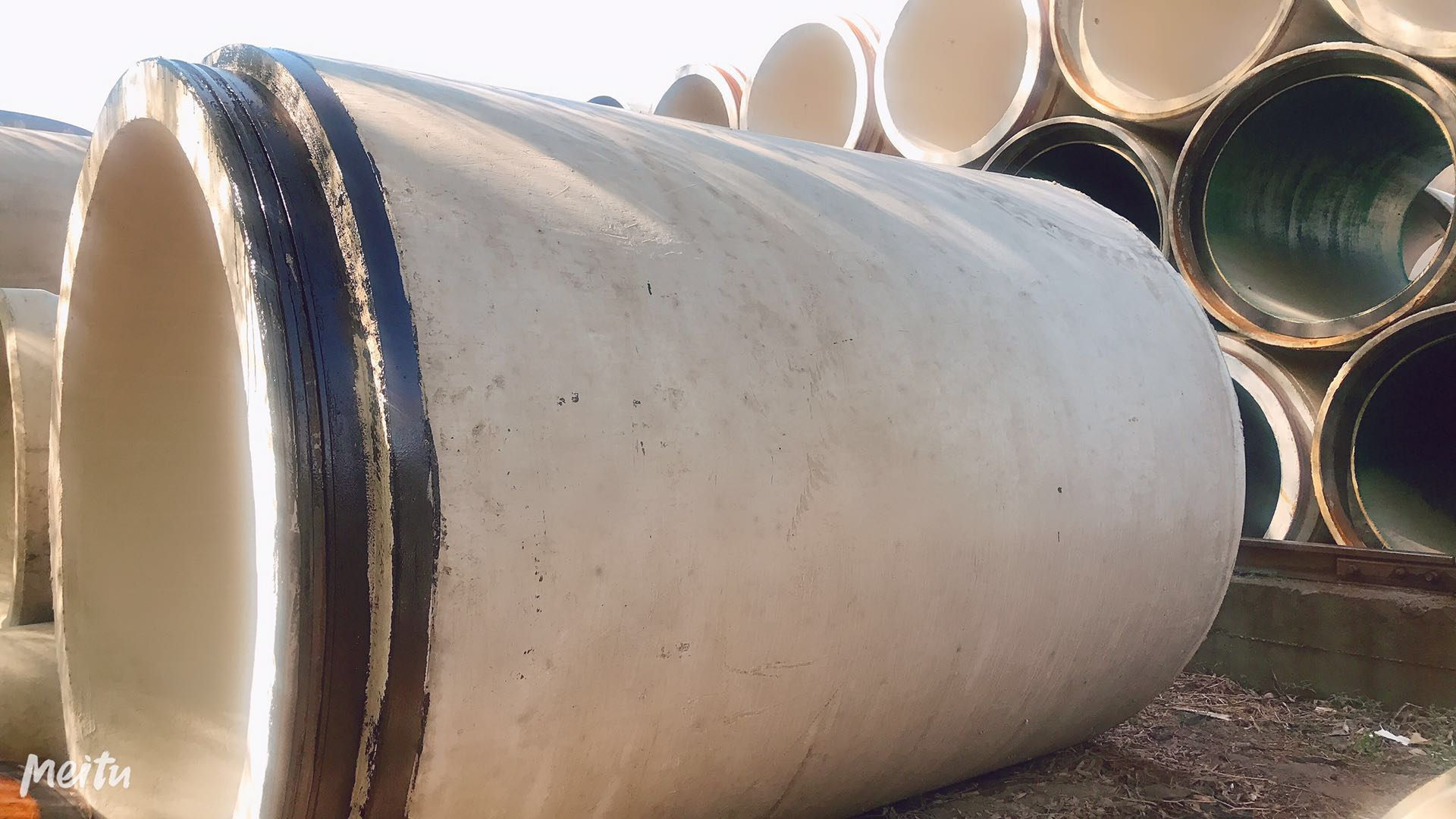m

13%
2020/10/28m

13%
2020/10/28m

13%
2020/10/28m

13%
2020/10/28m

13%
2020/10/28m

13%
2020/10/28m

13%
2020/10/28m

13%
2020/10/28m

13%
2020/10/28m

13%
2020/10/28m

13%
2020/10/28m

13%
2020/10/28m

13%
2020/10/28m

13%
2020/10/28m

13%
2020/10/28m

13%
2020/10/28m

13%
2020/10/28m

13%
2020/10/28m

13%
2020/10/28m

13%
2020/10/28m

13%
2020/10/28m

13%
2020/10/28m

13%
2020/10/28m

13%
2020/10/28m

13%
2020/10/28m

13%
2020/10/28m

13%
2020/10/28m

13%
2020/10/28m

13%
2020/10/28m

13%
2020/10/28m

13%
2020/10/28m

13%
2020/10/28m

13%
2020/10/28m

13%
2020/10/28m

13%
2020/10/28

• 1
• 2
• 3
• 4
• 5
• 6
• 18订阅中心广联达大数据